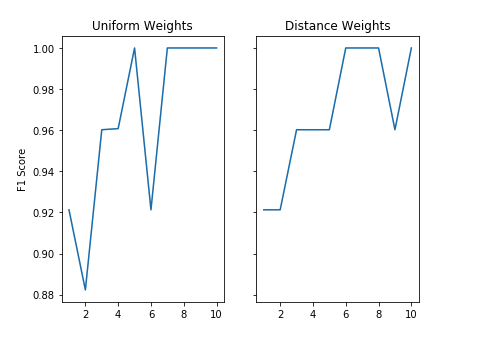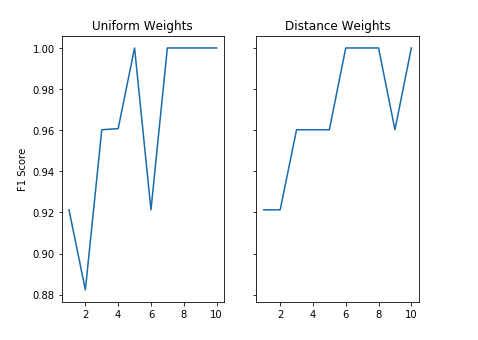# Introduction to Hyperparameter Optimization - Machine LearningThere are lots of knobs (a.k.a hyperparameters) we can turn when coming up with a Machine Learning model. in the script below, we take the well-known iris dataset, and play around with different hyperparameters.

First, a few notes:

In machine learning, we generally split our data into 3 sections

1. A training dataset
2. A dev dataset
3. A test dataset

We train the model on the training dataset, tune hyperparameters based on the dev dataset, and only run the test dataset when we're evaluating our model. Note that we don't want to tune hyperparameters based on the output of our test dataset in order to avoid overfitting both the test and training dataset

if you want to quickly iterate through many different hyperparameters, I recommend using a smaller subset of your data to allow for quick processing. Once some of the hyperparameters have been narrowed down, you can dedicate more time and computational resources to running the full training dataset and creating the model in your production workflow.

Without further ado, here is a hyperparameter optimization on K Nearest Neighbors using the corresponding classifier from the Python sklearn library

The below example uses Python 3.6 code.

First, we import our libraries:

```import pandas as pd
import numpy as np
from sklearn.model_selection import train_test_split
from sklearn.neighbors import KNeighborsClassifier
from sklearn.utils import shuffle
from sklearn.metrics import f1_score
import matplotlib.pyplot as plt
import random```

I set a random.seed() here to make results reproducible

`random.seed(123)`

In this example, we are building a dataframe which contains the iris dataset. However, for your own purposes, you can very well use your SQL output, which will get passed into the Periscope Python/R editor as a dataframe named df. Your final dataframe must have a list of features (these are the predictor components, you can think of these as your "X," and the corresponding label, you can think of this as your "y"). This dataframe below has 3 features: the sepal length, sepal width, and petal length. We also have a column, 'target,' which contains the name of the iris type.

```iris  = load_iris()
df = pd.DataFrame(data= np.c_[iris['data'], iris['target']],
columns= iris['feature_names'] + ['target'])
df = df.drop('petal width (cm)', axis = 1)```

Next, we shuffle the dataframe to ensure we are getting a representative sample of our data in the training, dev, and test dataset.

`df = shuffle(df, random_state = 300)`

Now,  we want to split our target column (y) from all of our features (our x values)

```df_features = df.drop('target', axis = 1)
features = df_features.values
target = df["target"].values```

Now we split our data into training, dev, and test datasets

```num_rows = df.shape
train_cutoff = int(num_rows * 0.6)
dev_cutoff = int(num_rows * 0.8)

features_train = features[:train_cutoff,:]
features_dev = features[train_cutoff:dev_cutoff,:]
features_test = features[dev_cutoff:,:]

target_train = target[:train_cutoff]
target_dev = target[train_cutoff:dev_cutoff]
target_test = target[dev_cutoff:]```

Now, we loop through all our hyperparameters. In this example, we are looping through all values of n_neighbors from 1 to 10. This determines how many of our "neighbors" we are using to classify a given point outside our training dataset. Additionally, we will compare the effectiveness of using "uniform" versus "distance" weights for our model.  Note that:

• A "uniform" weight takes a vote between the N closest neighbors of a point to classify it.
• A "distance" weight gives more importance to those neighbors that are closest to the point. For example, let's say our KNN is looking at n_neighbors of 5. if of the 5 closest neighbors, the closest of them all is Category A, that will be given more weight than the furthest of the 5 neighbors.

To evaluate the dataset, we will run a .predict() function on model using the dev dataset, and score the model using the F1 score (F1 is better at capturing false positives and false negatives, more info on this here). Note that you can achieve this logic with GridSearchCV as well

```all_k = range(1,11)
uniform = []
distance = []

# Looping through all values of k
for nbrs in all_k:
knn_uni = KNeighborsClassifier(n_neighbors = nbrs, weights = 'uniform')
knn_dist = KNeighborsClassifier(n_neighbors = nbrs, weights = 'distance')
pred_uni = knn_uni.fit(features_train, target_train).predict(features_dev)
pred_dist = knn_dist.fit(features_train, target_train).predict(features_dev)
f1_uni = f1_score(target_dev, pred_uni, average = 'macro')
f1_dist = f1_score(target_dev, pred_dist, average = 'macro')
uniform.append(f1_uni)
distance.append(f1_dist)```

Finally, we plot our results

```fig, (ax1, ax2) = plt.subplots(1, 2, sharey=True)
ax1.plot(all_k, uniform)
ax1.set_title('Uniform Weights')
ax1.set_ylabel('F1 Score')
ax2.plot(all_k, distance)
ax2.set_title('Distance Weights')

# Use Periscope to visualize a dataframe, text, or an image by passing data to periscope.table(), periscope.text(), or periscope.image() respectively.
periscope.image(fig)```Now we analyze the output. The number of neighbors used to generate the model is on the x axis, with the F1 score on the y axis. An F1 score closer to 1 is more desirable here. We see that there are more fluctuations in the uniform weights scoring compared to the distance weights. This is expected as we would anticipate the closest neighbors to be more informative when classifying an iris. Therefore, distance weights looks like a better option. Secondly, it looks like distance weights with 6-8 neighbors yield the highest F1 score. We would go on the lower end of our range here as n_neighbors of 6 is less computationally intensive than n_neighbors of 8 (we have fewer neighbors to account for when classifying each point).

Of course, we used a very very small dataset here, so we can expect the lines above to be smoother for a larger dataset.

Any other parameters you like to play around with for KNN?

Now that we have found our desired hyperparameters, let's run this on our test dataset! Let's put this in a Periscope view so it's easy to leverage this logic multiple times without rewriting code. See post here for further details!